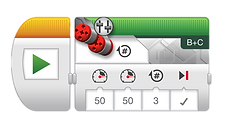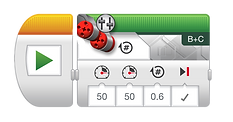top of page#### Robot Coding

Have you ever wondered how robots work?  Try this activity.  Watch the video on the right and answer the questions below.  Even if you don't have an lego robot with you can learn how it works.  You can also download this app if you have access to an iPad, however, you don't need any device to try this activity.   Have fun!

Getting Started1.  How far will the robot move when the wheels rotate one time?

(Hint: Review the video at 1:50)2.  How far will the robot move when the wheels rotate 3 times?

(Hint: Multiply the previous answer by 3)3.  How far will the robot move when the wheels rotate 6 times?

(Hint: Multiply the previous answer by 2)

How can you make the robot turn?

Watch this video and answer the following questions.

Turning4.  How far will the robot turn when one wheel rotates 1.2 times?

(Hint: Review the video about turning)5.  How far will the robot turn when the wheel rotates  0.6 times?

(Hint: How far did the robot go when the wheels rotated 6 times?  Divide that answer by 10)

Final Question

7.  Based on what you've learned so far, can you predict all three movements this robot will make?